×
Get Full Access to Stats: Data And Models - 4 Edition - Chapter 5 - Problem 20e
Get Full Access to Stats: Data And Models - 4 Edition - Chapter 5 - Problem 20e

×

# Windy In the last chapter, we looked at three outliersISBN: 9780321986498 70

## Solution for problem 20E Chapter 5

Stats: Data and Models | 4th Edition

• Textbook Solutions
• 2901 Step-by-step solutions solved by professors and subject experts
• Get 24/7 help from StudySoup virtual teaching assistantsStats: Data and Models | 4th Edition

4 5 1 289 Reviews
24
0
Problem 20E

Problem 20E

Windy In the last chapter, we looked at three outliers arising from a plot of Average Wind Speed by Month in the Hopkins Forest. Each was associated with an unusually strong storm, but which was the most remarkable for its month?

Here are the summary statistics for each of those three months:The outliers had values of 6.73 mph, 3.93 mph, and 2.53 mph, respectively.

a) What are their z-scores?

b) Which was the most extraordinary wind event?

Step-by-Step Solution:
Step 1 of 2

Problem 20E

Windy In the last chapter, we looked at three outliers arising from a plot of Average Wind Speed by Month in the Hopkins Forest. Each was associated with an unusually strong storm, but which was the most remarkable for its month?

Here are the summary statistics for each of those three months: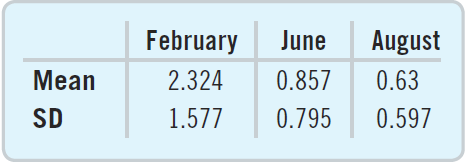The outliers had values of 6.73 mph, 3.93 mph, and 2.53 mph, respectively.

a) What are their z-scores?

b) Which was the most extraordinary wind event?

Step by step solution:

Step1:

Given values,

February:

Mean,Standard deviation,Outlier,June:

Mean,Standard deviation,Outlier,August:

Mean,Standard deviation,Outlier,We know that the equation for z-score is,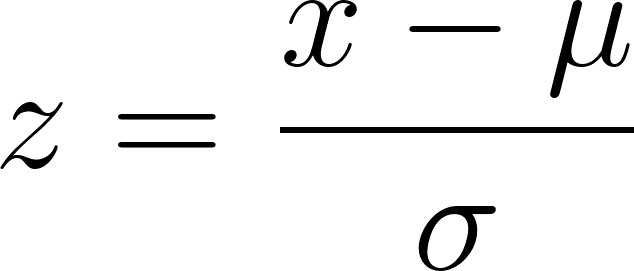Now, let’s find the z-scores

February: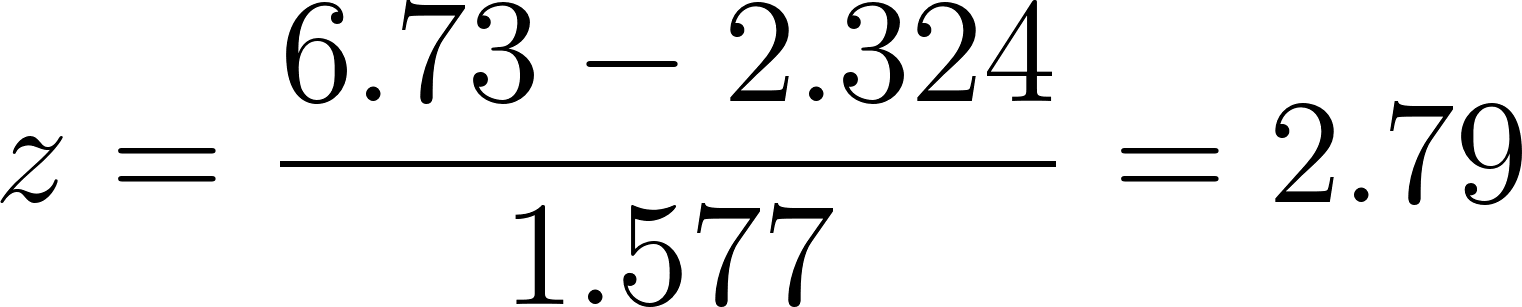For June: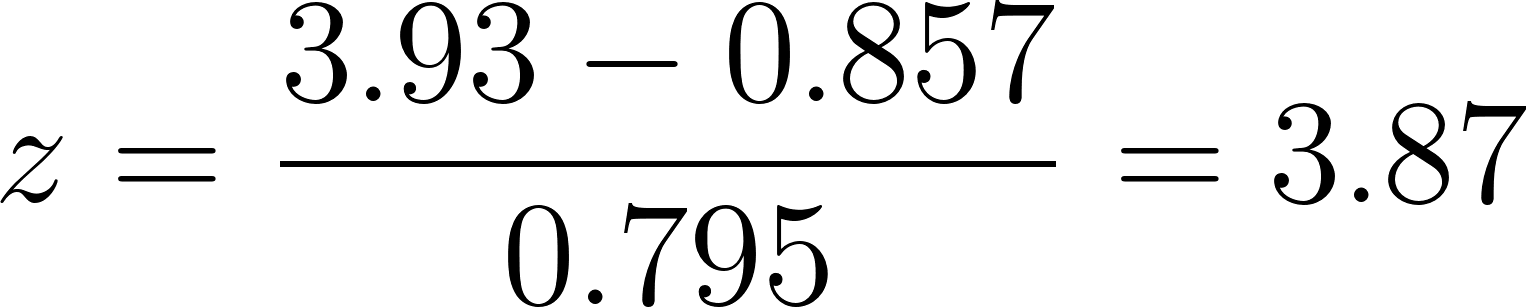For August: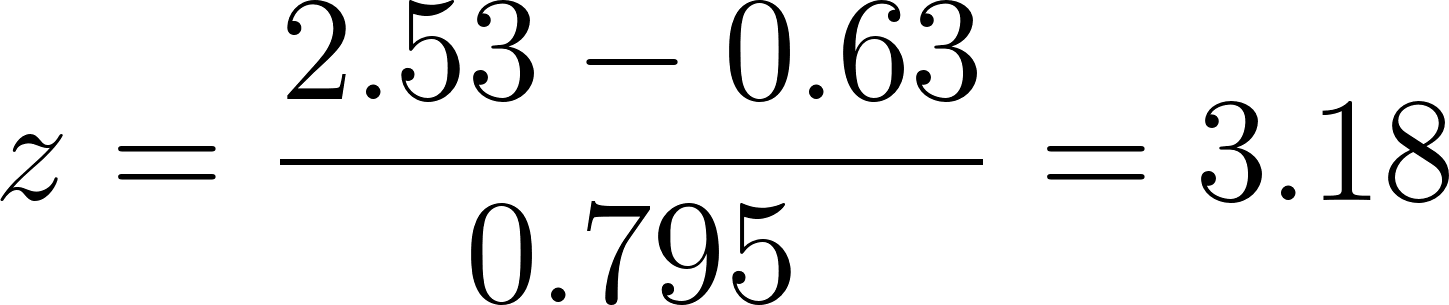Step 2 of 2

##### ISBN: 9780321986498

Unlock Textbook Solution